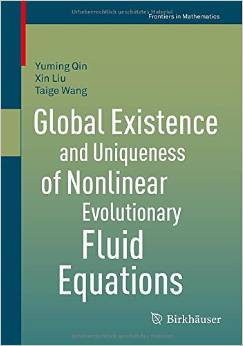# Global Existence And Uniqueness Of Nonlinear Evolutionary Fluid Equations by Xin Liu / 2015 / English / PDF

This book presents recent results on nonlinear evolutionary fluid equations such as the compressible (radiative) magnetohydrodynamics (MHD) equations, compressible viscous micropolar fluid equations, the full non-Newtonian fluid equations and non-autonomous compressible Navier-Stokes equations. These types of partial differential equations arise in many fields of mathematics, but also in other branches of science such as physics and fluid dynamics. This book will be a valuable resource for graduate students and researchers interested in partial differential equations, and will also benefit practitioners in physics and engineering.

views: 622